The magnitude of electric field E in the annular region of a charged cylindrical capacitor

(1) Is same throughout

(2) Is higher near the outer cylinder than near the inner cylinder

(3) Varies as 1/r, where r is the distance from the axis

(4) Varies as 1/r2, where r is the distance from the axis

Concept Questions :-

Electric field
High Yielding Test Series + Question Bank - NEET 2020

Difficulty Level:

A metallic solid sphere is placed in a uniform electric field. The lines of force follow the path(s) shown in figure as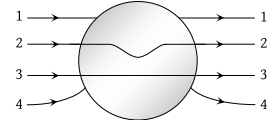(1) 1

(2) 2

(3) 3

(4) 4

Concept Questions :-

Electric field
High Yielding Test Series + Question Bank - NEET 2020

Difficulty Level:

The figure shows some of the electric field lines corresponding to an electric field. The figure suggests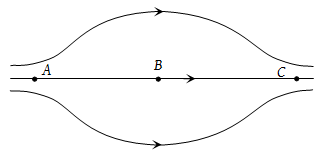(1) EA > EB > EC

(2) EA = EB = EC

(3) EA = EC > EB

(4) EA = EC < EB

Concept Questions :-

Electric field
High Yielding Test Series + Question Bank - NEET 2020

Difficulty Level:

A hollow insulated conducting sphere is given a positive charge of 10μC. What will be the electric field at the centre of the sphere if its radius is 2 meters

(1) Zero

(2) 5μ Cm–2

(3) 20μ Cm–2

(4) 8μ Cm–2

Concept Questions :-

Electric field
High Yielding Test Series + Question Bank - NEET 2020

Difficulty Level:

An electron of mass me initially at rest moves through a certain distance in a uniform electric field in time t1. A proton of mass mp also initially at rest takes time t2 to move through an equal distance in this uniform electric field. Neglecting the effect of gravity, the ratio of t2/ t1 is nearly equal to

(1) 1

(2) ${\left({m}_{p}/{m}_{e}\right)}^{1/2}$

(3) ${\left({m}_{e}/{m}_{p}\right)}^{1/2}$

(4) 1836

Concept Questions :-

Electric field
High Yielding Test Series + Question Bank - NEET 2020

Difficulty Level:

Point charges +4q, –q and +4q are kept on the x-axis at points x = 0, x = a and x = 2a respectively, then

(1) Only -q is in stable equilibrium

(2) None of the charges are in equilibrium

(3) All the charges are in unstable equilibrium

(4) All the charges are in stable equilibrium

Concept Questions :-

Coulomb's law
High Yielding Test Series + Question Bank - NEET 2020

Difficulty Level:

Three identical point charges, as shown are placed at the vertices of an isosceles right angled triangle. Which of the numbered vectors coincides in direction with the electric field at the mid-point M of the hypotenuse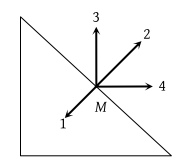(1) 1

(2) 2

(3) 3

(4) 4

High Yielding Test Series + Question Bank - NEET 2020

Difficulty Level:

Figures below show regular hexagons, with charges at the vertices. In which of the following cases the electric field at the centre is not zero?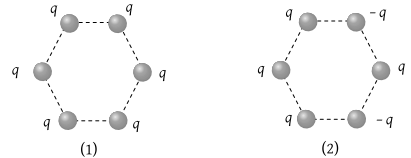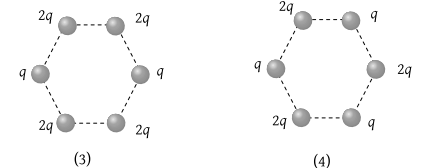(1) 1

(2) 2

(3) 3

(4) 4

Concept Questions :-

Electric field
High Yielding Test Series + Question Bank - NEET 2020

Difficulty Level:

An electron enters an electric field with its velocity in the direction of the electric lines of force. Then

(1) The path of the electron will be a circle

(2) The path of the electron will be a parabola

(3) The velocity of the electron will decrease

(4) The velocity of the electron will increase

Concept Questions :-

Electric field
High Yielding Test Series + Question Bank - NEET 2020

Difficulty Level:

The dimension of (1/2) : permittivity of free space; E: electric field) is

(1) MLT–1

(2) ML2L–2

(3) ML–1T–2

(4) ML2T–1

Concept Questions :-

Electric field